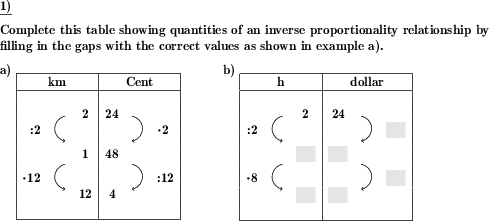Custom math worksheets at your fingertips# Details for problem "Inverse proportionality - complete table"

Quickname: 6517

Elementary School, Primary School, Junior High School, Middle School, High School.

## Summary

Apply the rule of three for an inverse proportionality relationship and fill in missing values in a table.

## Example## Description

A rule of three problem for an inverse proportionality relationship has to be solved. It is presented in the form of a table. To solve the problem, complete the table by filling in the missing values.

The table is provided with values that will be sufficient to solve the problem. The difficulty of the problem is depending on the values that are provided and the values that have to be calculated and derived from others.

The values of the tables are as follows:

 argument x1>1 value y1 divisor on left = factor on right argument x2=1 value y2 factor on left = divisor on right argument x3≠x1 value y3

The level of difficulty can be chosen from five levels. The level of difficulty determined what values will be provided with the table:

Level 1
Argument x1, value y1, divisor on left, argument x2, argument x3 are given. The solution is straightforward.
Level 2
Argument x1 and value y1 plus others are given as necessary so that values have to be derived in a way that the table can be filled from top to bottom down the table rows.
Level 3
Argument x1, value y1 are provided together with x3 on the left or value y3 on the right, so value will have to be derived in a bottom to top way too.
Level 4
Only one of argument x1 or value y1 are given, plus others as necessary to solve the problem.
Level 5
Neither argument x1 nor value y1 are given, the derivation of values from others will have to be performed in a bottom up fashion.

The number of problems is selectable. The first problem may be presented as a sample problem, showing the complete table with all values. A number range can be chosen for the numbers appearing in the table.

Download free printable worksheets for this math problem here. The worksheet contains the problems only, the solution sheet includes the answers. Just click on the respective link.

•Worksheet 1Solution sheet with answers
•Worksheet 2Solution sheet with answers
•Worksheet 3Solution sheet with answers

If you can not see the solution sheets for download, they may be filtered out by an ad blocker that you may have installed. If this is the case, please allow ads for this page and reload the page. The solution sheets will then reappear.

• Do these sample worksheets do not really fit?
• Do you need more math worksheets, with a different level of difficulty?
• Would you like to combine different problems on a worksheet and adjust them to your needs?
• As a teacher, you can put together your own worksheets using the automatically generated math problems provided.
With a free initial credit, you can start creating your own math worksheets in a few minutes.

You can try it for free! Register here, to create custom worksheets now!

## Customization options for this problem

Parameter
Possible values
Number of problems
1, 2, 3, 4, 5, 6, 7, 8
Number range
100, 200, 500, 1000, 10000, 100000
Level of difficulty
1, 2, 3, 4, 5
Sample problem
Yes, No

## Similar problems

Remark
Description
Similar problem for directly proportional relationships
Apply the rule of three for a direct proportionality relationship and fill in missing values in a table.
In the form of a word problem
Word problems that can be solved by applying the rule of three.